# Pitt Quantum Repository

Database of molecular quantum calculations

The Pitt Quantum Repository is a database and website of molecular quantum calculations, including visualization

## Examples

### Basic Examples

Retrieve the resource:

 In:=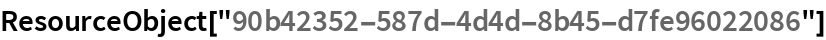Out=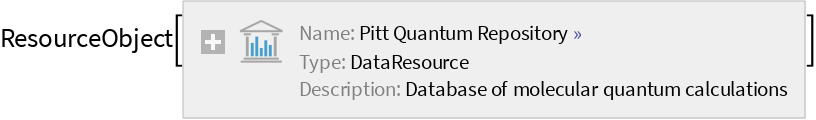Retrieve the default content:

 In:=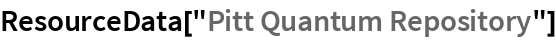Out=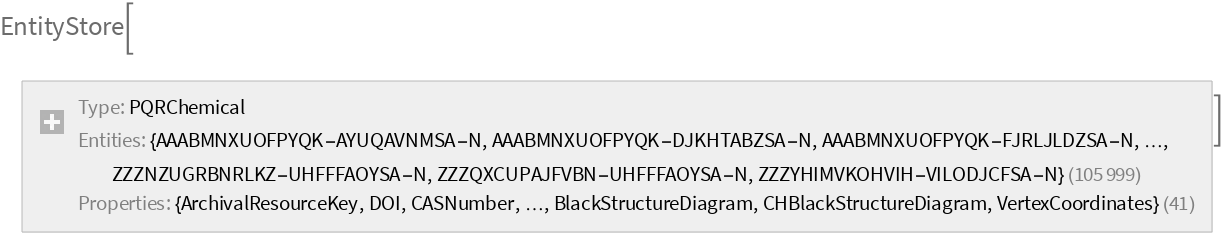Register the EntityStore:

 In:=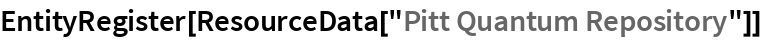Out=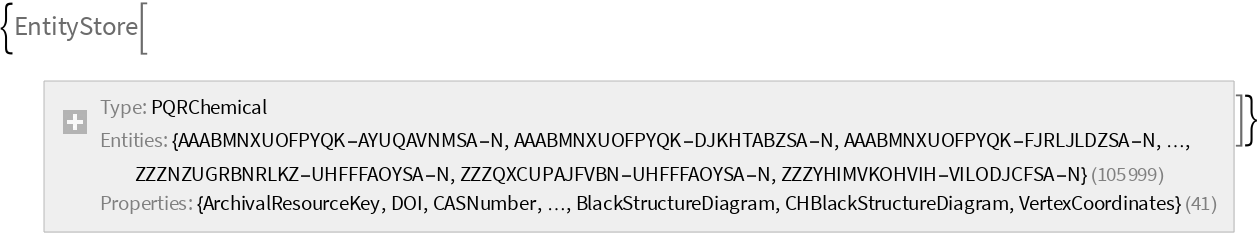### Analysis

Find the total number of entities:

 In:=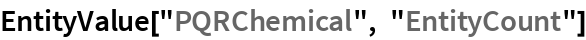Out=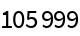View random entities:

 In:=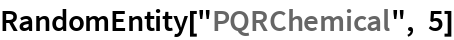Out=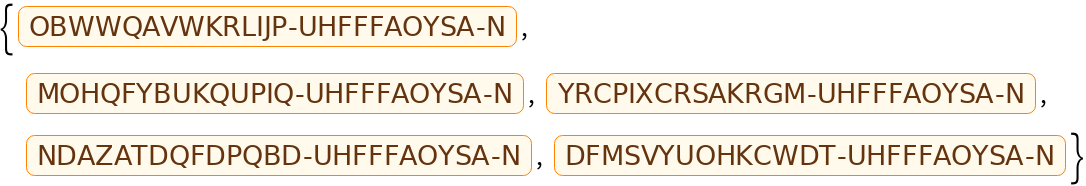Get the available properties for the entity store:

 In:=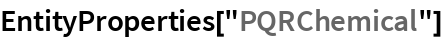Out=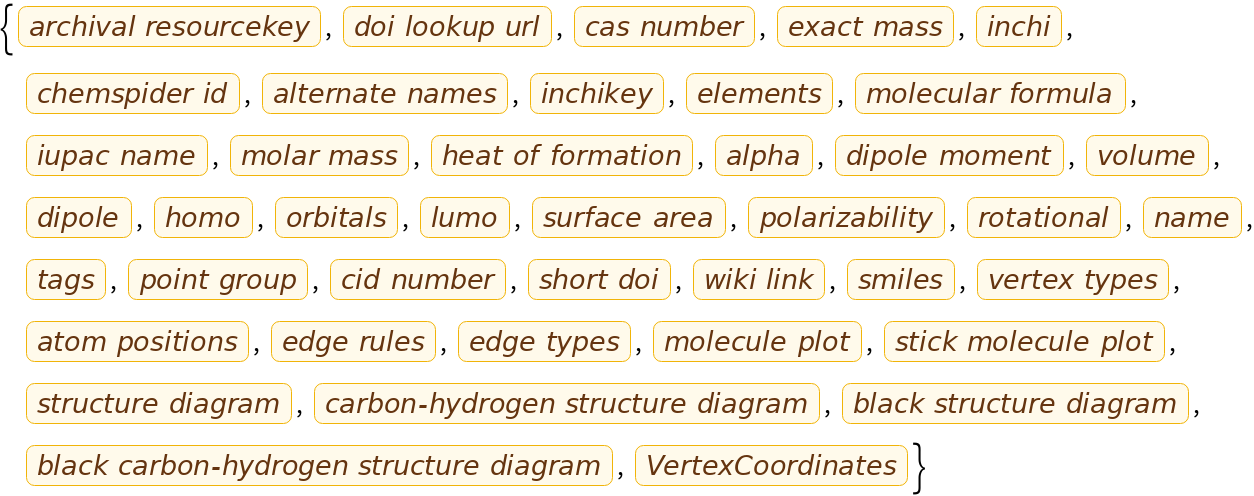Retrieve specific property values:

 In:=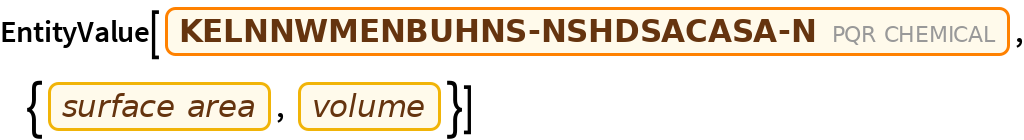View all properties from an Entity in a Dataset:

 In:=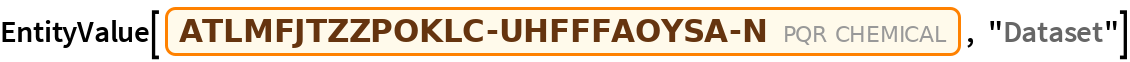Out=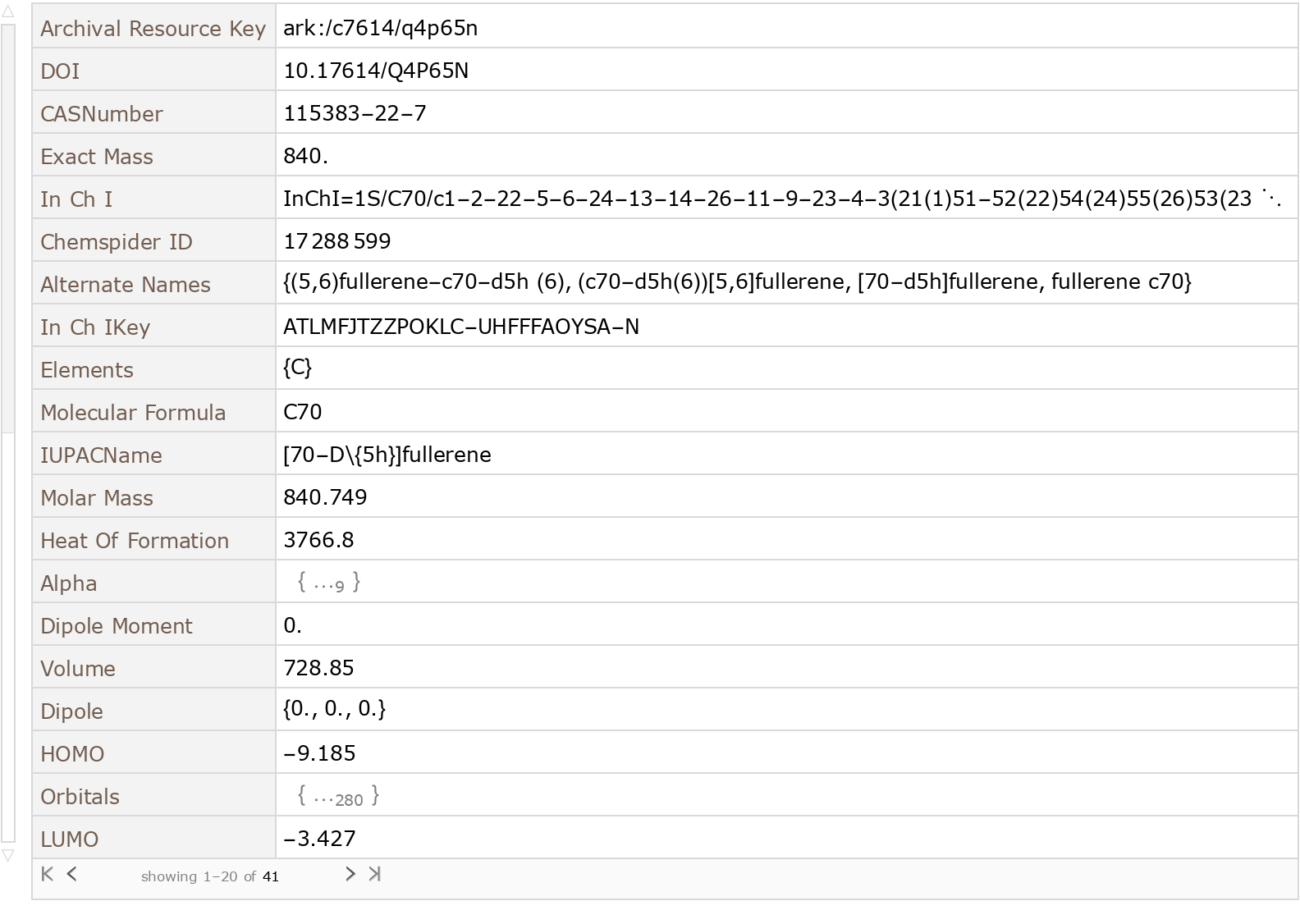### Visualization

 In:=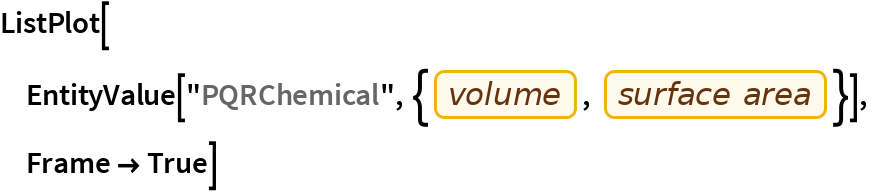Out=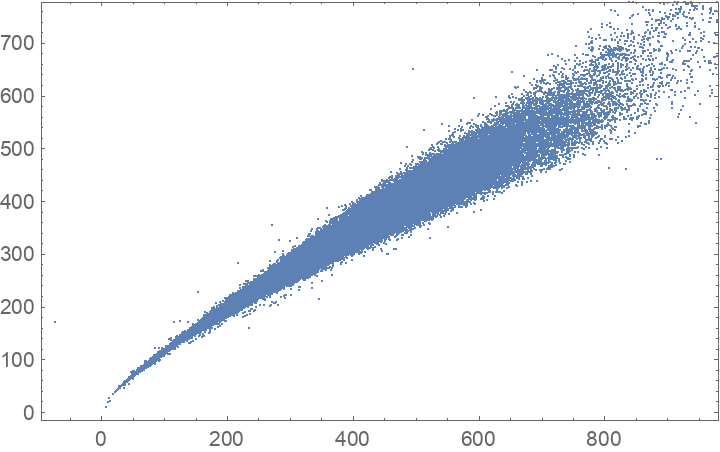Use the Wolfram Language Graph functionality to find the number of distinct rings with fewer than 8 atoms in each molecule, then display this in a histogram:

 In:=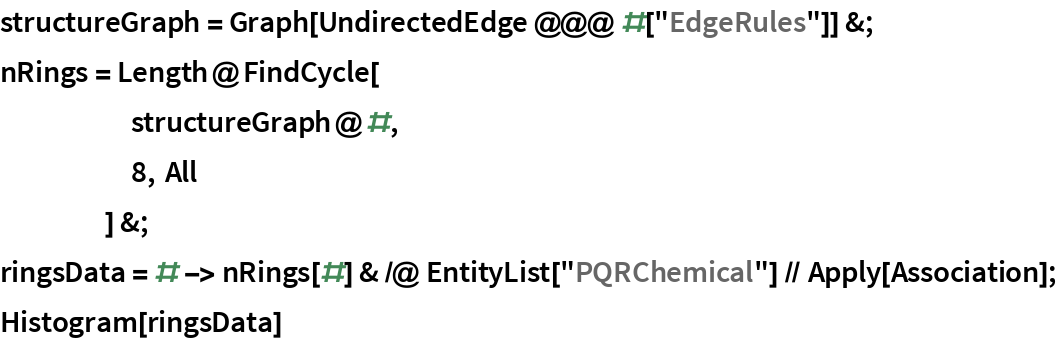Out=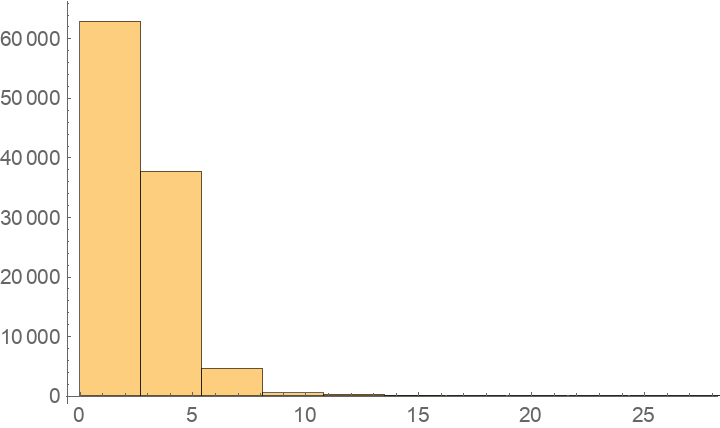View the 3D structure for the entities with the largest number of rings:

 In:=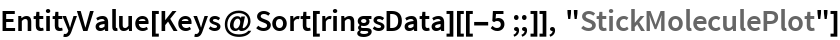Out=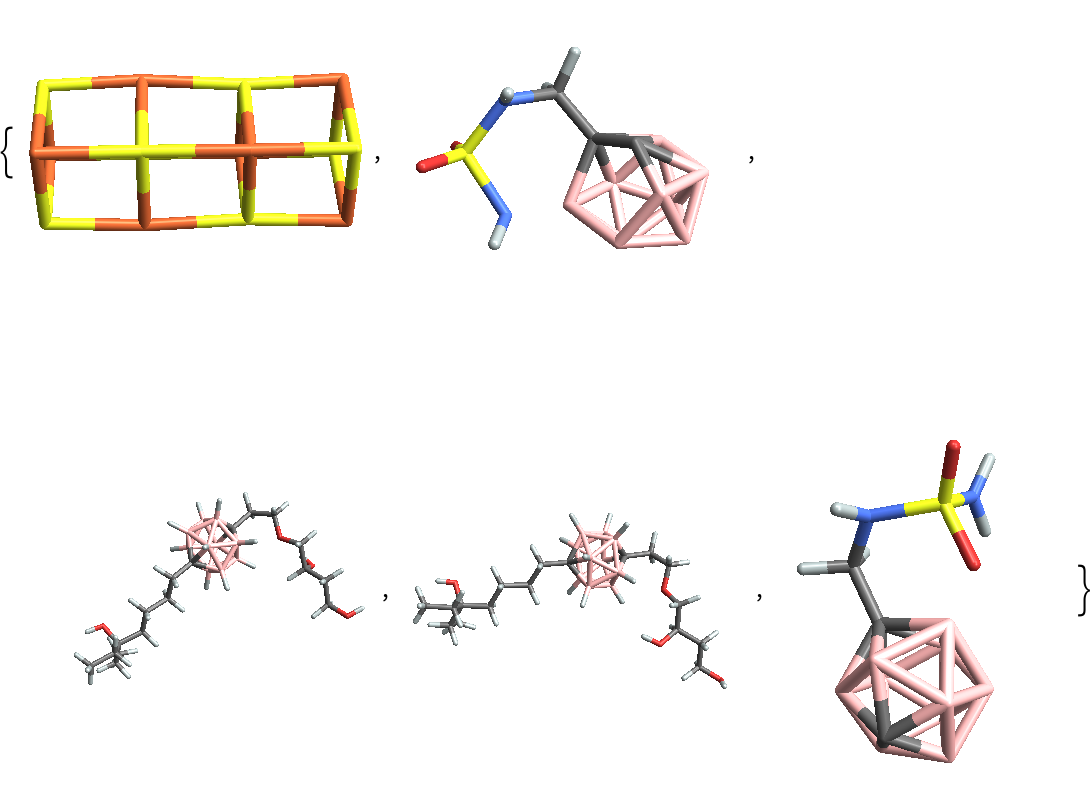Wolfram Research, "Pitt Quantum Repository" from the Wolfram Data Repository (2017) https://doi.org/10.24097/wolfram.83637.data